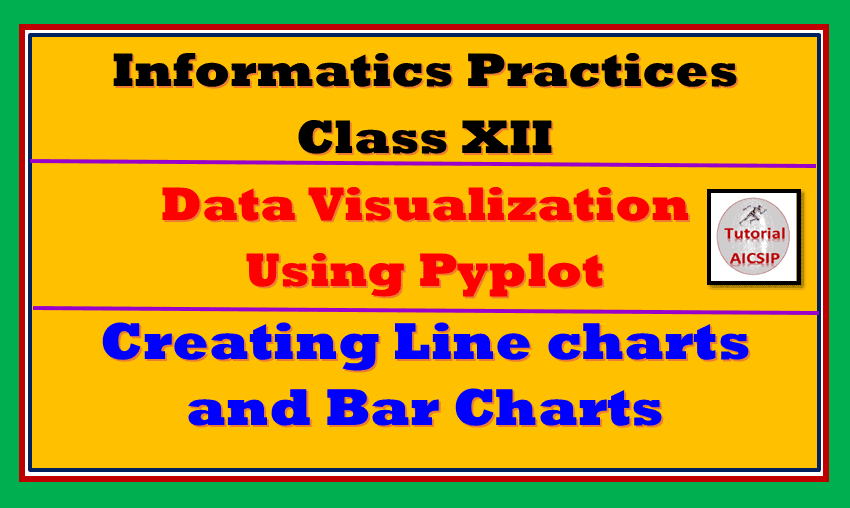In this article, you are going to learn about Data Visualization Class 12 IP. I am going to explain creating bar chart and line charts in this article.

Topics Covered

## Data Visualization Class 12 IP

Let us start the article Comprehensive notes Data Visualization Class 12 IP with few quotes. Read the following quotes:
Visualization gives you answers to questions you didn’t know you had.. – Ben Schneiderman
An editorial approach to visualization design requires us to take responsibility to filter out the noise from the signals, identifying the most valuable, most striking or most relevant dimensions of the subject matter in question. – Andy Kirk
Data visualization doesn’t live in an ethereal dimension, separated from the data. When there’s a large number of pie-charts in a report or a presentation, there is something wrong in the organization, and it’s not the pie. A pie chart is a potential symptom of lack of data analysis skills that have to be resolved. – Jorge Camoes

Pictures playing an important role in representing data. As we all are aware that pictures giving a more and more clear understanding of any kind of data or complex problems. Some of the images help to understand the structure or patterns of data flow and execution.

Before going ahead, If you missed the notes on data frames check out our main page of the Informatics Practices class XII portion.

In the next section of Comprehensive notes Data Visualization Class 12 IP we are going to discuss basic components of graphs.

Basic components of Graph

A graph has the following basic components:
1. Figure or chart area:  The entire area covered by the graph is known as a figure. It can be also considered as a canvas or chart area also.
2. Axis: These are the number of lines generated on the plot. Basically, there are two axis X and Y-axis.
3. Artist: The components like text objects, Line 2D objects, collection objects, etc.
4. Titles: There are few titles involved with your charts such as Chart Title, Axis title, etc.
5. Legends: Legends are the information that represents data with lines or dots.

After getting familiar with parts of the graph, let me introduce matplotlib for Comprehensive notes Data Visualization Class 12 IP.

## matplotlib Introduction

Python supports a variety of packages to handle data. Matplotlib is also one of the most important packages out of them. It is a low-level library integrated with Matlab like interface offers few lines of code and draw graphs or charts. It has modules such as a pyplot to draw and create graphs.

In the next section of Comprehensive notes Data Visualization Class 12 IP you will known sthe steps required to create a chart.

## Steps – how to create graphs using matplotlib

The following are basic steps to create a chart.

### Step 1 Installation of matplotlib

Install matplotlib by following these simple steps:

Step 1: Open cmd from the start menu
Step 2: Type pip install matplotlib

### Step 2 import module

Import matplotlib.pylot using import command in the following two ways:

1. Without instance: import matplotlib.pyplot
2. With instance: import matplotlib.pyplot as mpp

### Step 3 Choose desired plot type (graph type)

In this step, select your desired chart type for plotting. For example, line chart

### Step 4 Give proper labels to axis, categories

A graph is made up of two-axis i.e. X and Y-axis. In this step label them as per the need as well as apply proper labels for categories also.

### Step 5 Add data points

The next point is to add data points. Data points depict the point on the plot at a particular place.

### Step 6 Add more functionality like colours, sizes etc

To make your graphs more effective and informative use different colours and different sizes.

The common method used to plot a chart is plot().

In the next section of Comprehensive notes Data Visualization Class 12 IP, you will learn about pyplot package.

## The PyPlot package

The Pyplot package provides an interface to plot the graph automatically as per the requirements. You just need to provide accurate values for axes, categories, labels, title, legend, and data points.

Matplotlib provides the following types of graphs in python:
• Line plot
• Bar graph
• Histogram
• Pie chart
• Scatter chart
In the next section of Comprehensive notes Data Visualization Class 12 IP you will learn creating a line chart or plotting lines.

## Creating a Line chart or Plotting lines

To create a line chart following functions are used:
• plot(x,y,color,others): Draw lines as per specified lines
• xlabel(“label”): For label to x-axis
• ylabel(“label”): For label to y-axis
• title(“Title”): For title of the axes
• legend(): For displaying legends
• show() : Display the graph

Now observe the following code:

```import matplotlib.pyplot as mpp
mpp.plot(['English','Maths','Hindi'],[88,90,94],'Red')
mpp.xlabel('Subjects')
mpp.ylabel('Marks')
mpp.title('Progress Report Chart')
mpp.show()

```
Output:

In the above code, 3 subject marks are plotted on the figure. The navigation toolbar helps to navigate through the graph. Now observe the following code for plotting multiple lines on the graph.
```import matplotlib.pyplot as mpp
o=[5,10,15,20]
r_india=[30,80,120,200]
mpp.plot(o,r_india,'Red')
r_aust=[25,85,100,186]
mpp.plot(o,r_aust,'Yellow')
mpp.xlabel('Runs')
mpp.ylabel('Overs')
mpp.title('Match Summary')
mpp.show()```
Output:

So now you understand how to plot lines on the figure. You can change the colour using abbreviations and line style by using the linestyle parameter also. Just do the following changes in above-given code and see the output:

mpp.plot(o,r_india,’m’,linestyle=’:’)
mpp.plot(o,r_aust,’y’,linestyle=’-.’)

## Bar Graph

The bar graph represents data in horizontal or vertical bars. The bar() function is used to create bar graph. It is most commonly used for 2D data representation. Just have a look at the following code:
```import matplotlib.pyplot as mpp
overs=[5,10,15,20]
runs=[30,80,120,200]
mpp.bar(runs,overs,width=30, label='Runs',color='r')
mpp.xlabel('Runs')
mpp.ylabel('Overs')
mpp.title('Match Summary')
mpp.legend()
mpp.show()```
Output:

I hope you are enjoying this article Comprehensive notes Data Visualization Class 12 IP, kindly share your view in the comment section.